# 网络流量预测入门（一）之RNN 介绍

## RNN简介

RNN全称Recurrent Neural Network ，中文名为循环神经网络（亦或称递归神经网络）。相信大家在看这篇博客之前都已经简单的了解过RNN。将RNN说的简单一点，就是进行预测（或者回归）的时候，不仅要考虑到当前时刻的输入，还要考虑上一个时刻的输入（甚至有些RNN的变种还会考虑未来的情况）。换句话说，预测的结果不仅与当前状态有关，还与上一个时刻的状态有关。

RNN用于处理时序信息 。而在传统的神经网络中，我们认为输入的 $x_1,x_2,x_3$，是相互独立的：比如说在Iris分类中，我们认为鸢尾花的长宽是独立的，之间不存在前后序列逻辑关系。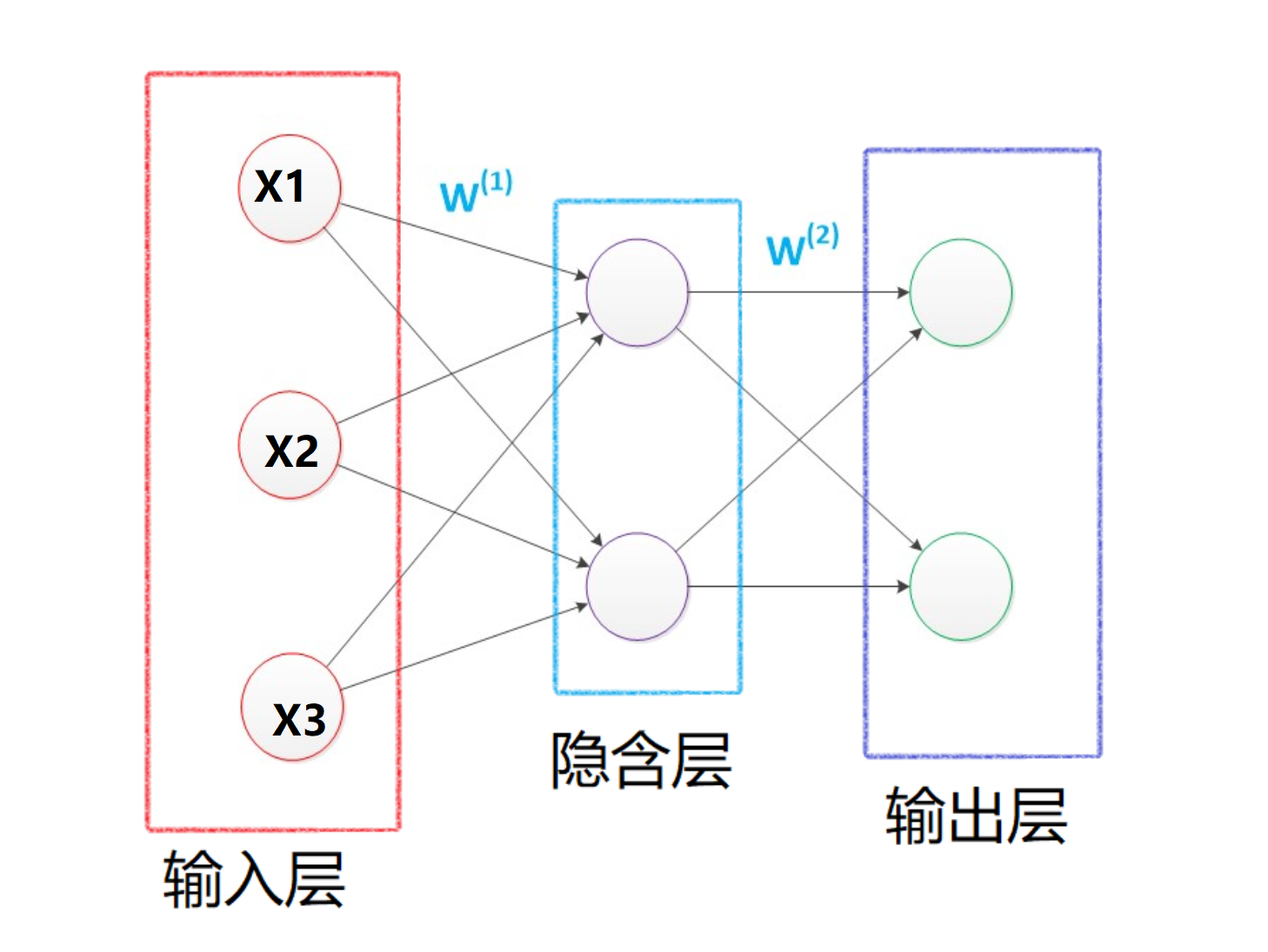• 若用前馈网络：网络训练过程相当于不用转盘，而是徒手将各个角度捏成想要的形状。不仅工作量大，效果也难以保证。
• 若用递归网络（RNN）：网络训练过程相当于在不断旋转的转盘上，以一种手势捏造所有角度。工作量降低，效果也可保证。

## RNN 结构

RNN的原理图，我们最多见的便是如左图所示，但是实际上将它展开，便是如下右图所示。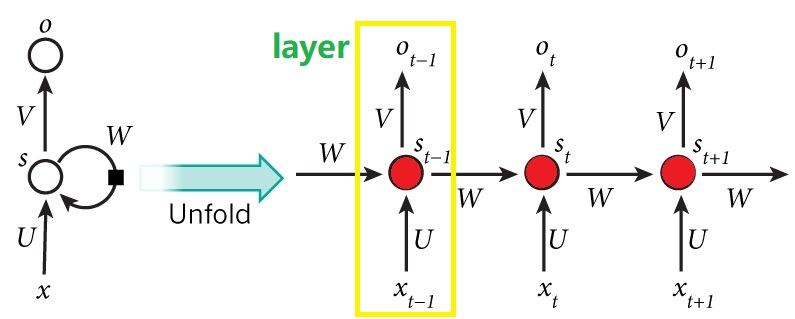1. 在RNN中，我们可以将黄框称之为一个layer，所有的layer的参数在一个batch中是相同的（参数共享），也就是说，上图中的 $U,W,V$ 等参数在某个batch全部相同。（通过一个batch的训练之后，经过反向传播，参数会发生改变）

2. Layer的层数根据自己的需要来定，举个例子，比如说我们分析的句子是5个单词构成的句子，那么layer的层数便是5，每一个layer对应一个单词。

3. 上图既有多个输入$X_{t-1},X_{t},X_{t+1}$ ， 也可以有多个输出$O_{t-1},O_{t},O_{t+1}$ , 但是实际上输出可以根据实际的需要而定，既可以为多个输出，也可以只有一个输出，有如下几种：

Type of RNN Illustration Example
One-to-one $T_x=T_y=1$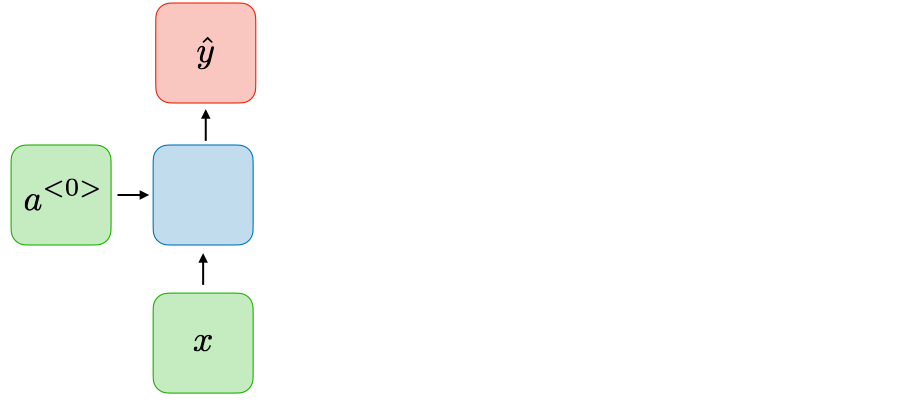Traditional neural network
One-to-many $T_x=1, T_y>1$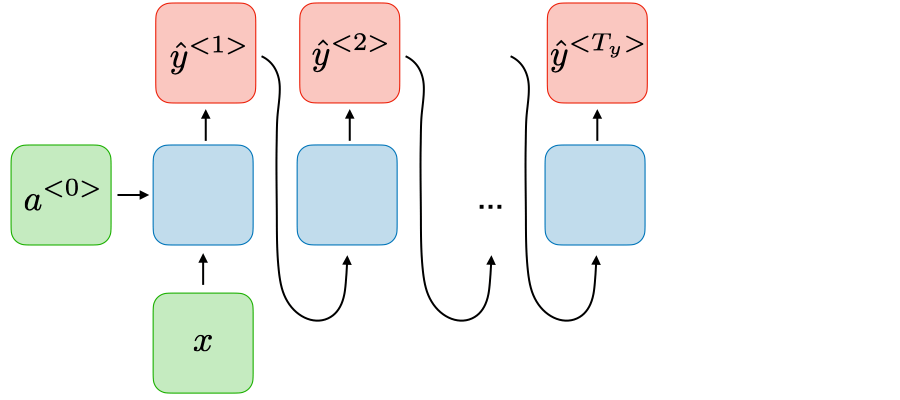Music generation
Many-to-one $T_x>1, T_y=1$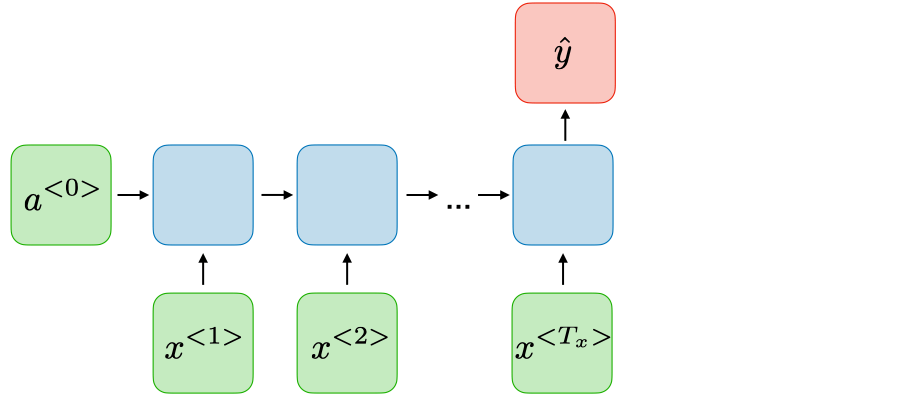Sentiment classification
Many-to-many $T_x=T_y$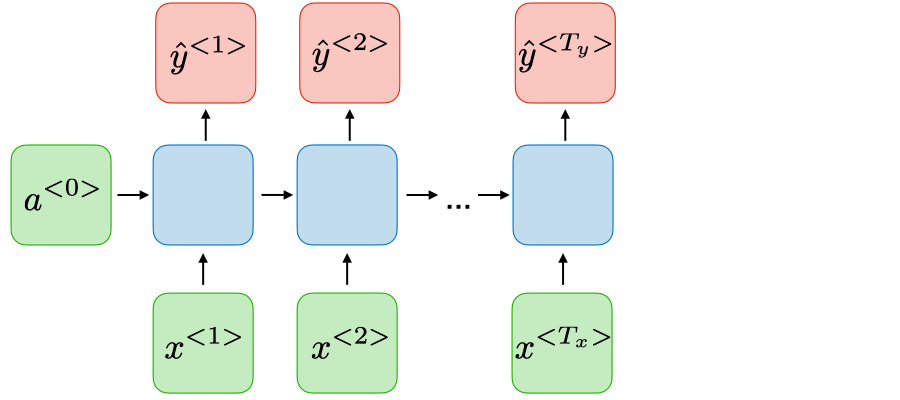Name entity recognition
Many-to-many $T_x\neq T_y$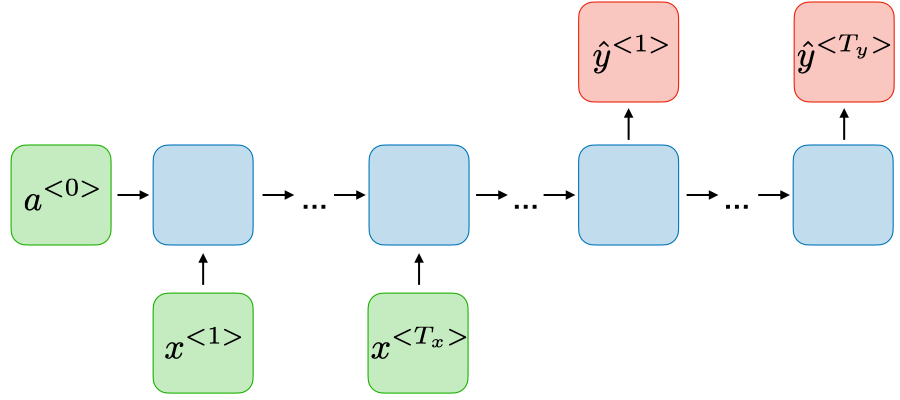Machine translation

Gif图如下所示：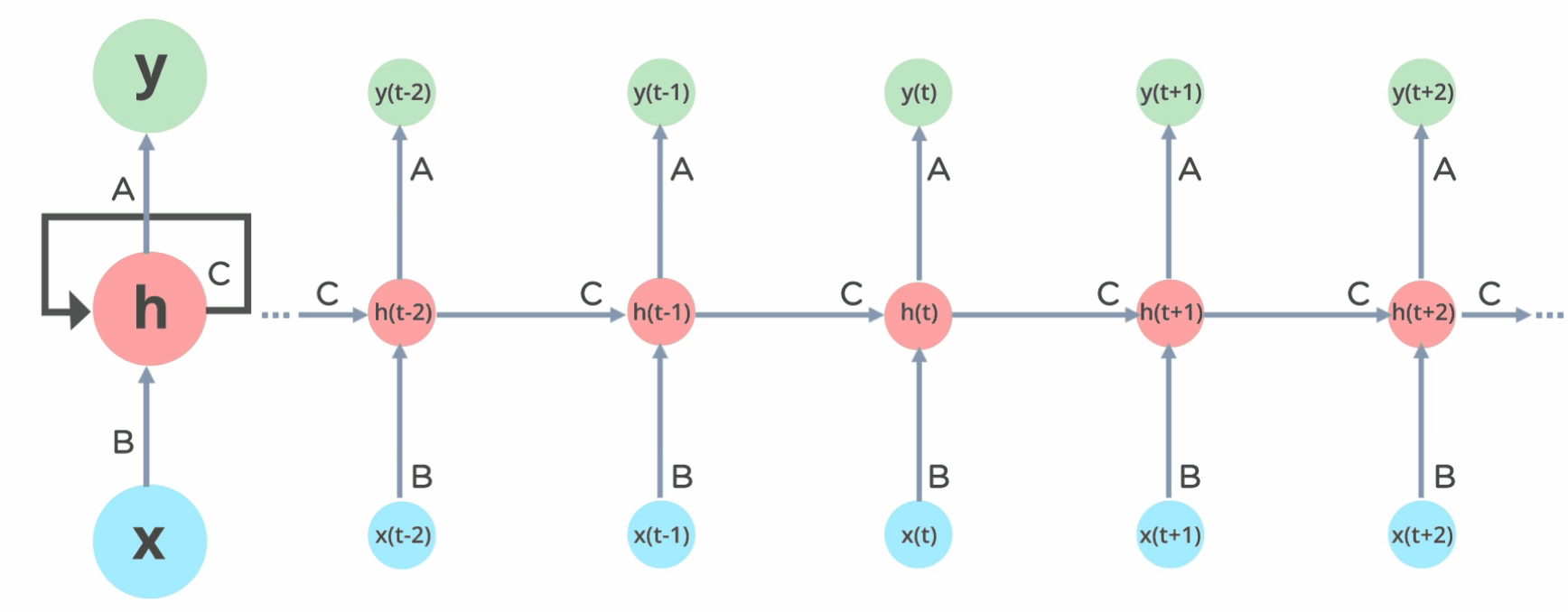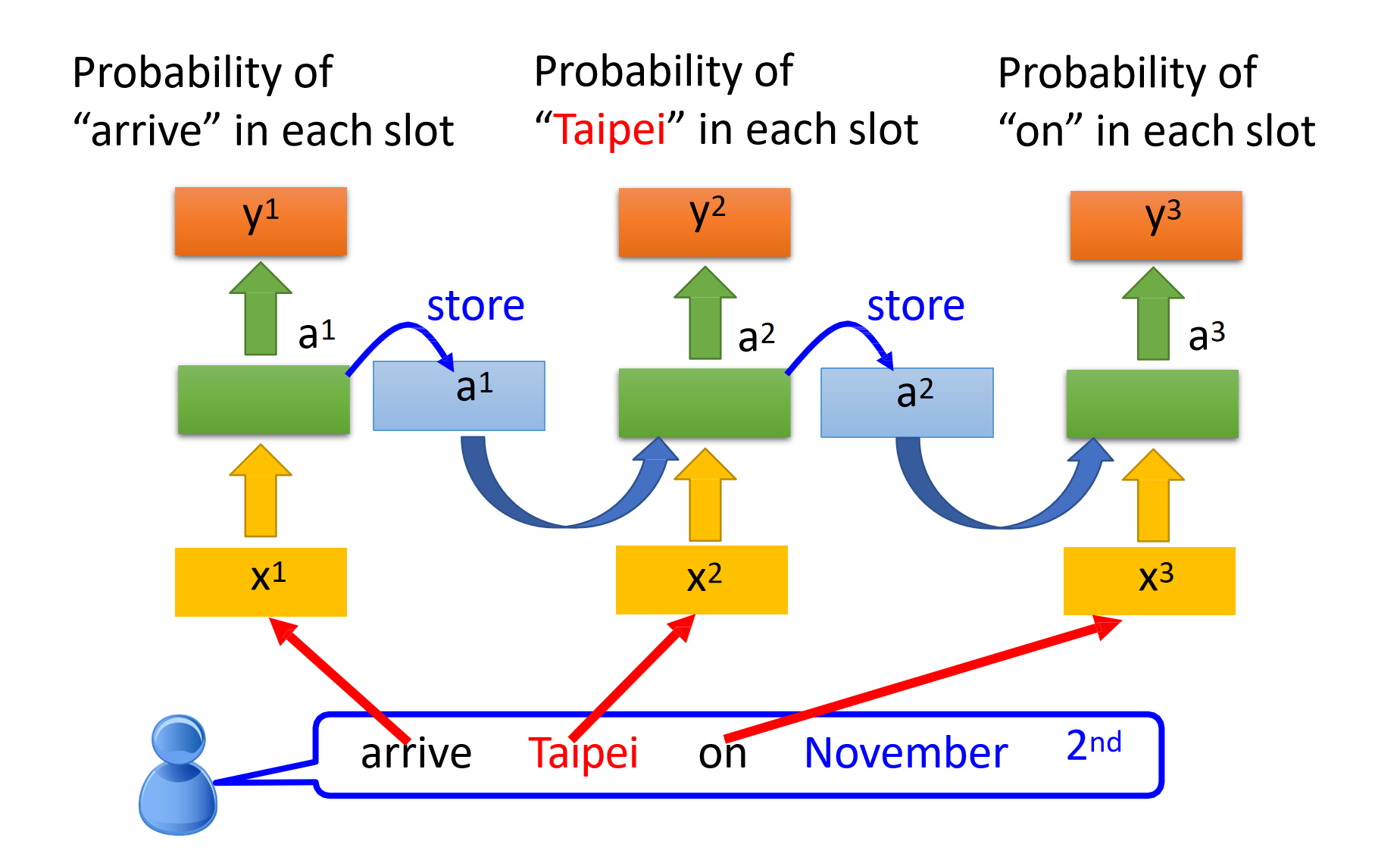## RNN原理

### 结构原理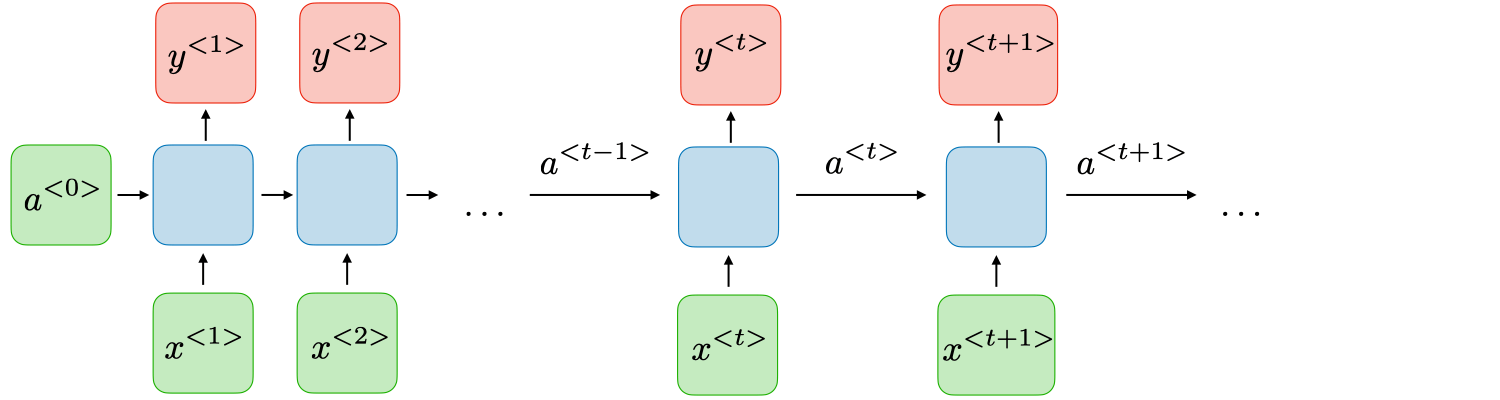$a^{<t>}$$y^{<t>}$ 的表达式如下所示：

$a^{<t>}=g_{1}\left(W_{a a} a^{<t-1>}+W_{a x} x^{<t>}+b_{a}\right) \quad \text { and } \quad y^{<t>}=g_{2}\left(W_{y a} a^{<t>}+b_{y}\right)$

• $W_{a x}, W_{a a}, W_{y a}, b_{a}, b_{y}$ 在时间上是共享的：也就是说，在一个batch中，无论是哪一个layer，其$W_{a x}, W_{a a}, W_{y a}, b_{a}, b_{y}$都是相同的(shared temporally)。当然，经过一个batch的训练之后，其值会因为反向传播而发生改变。

• $g_{1}, g_{2}$ 皆为激活函数（比如说tanh，sigmoid）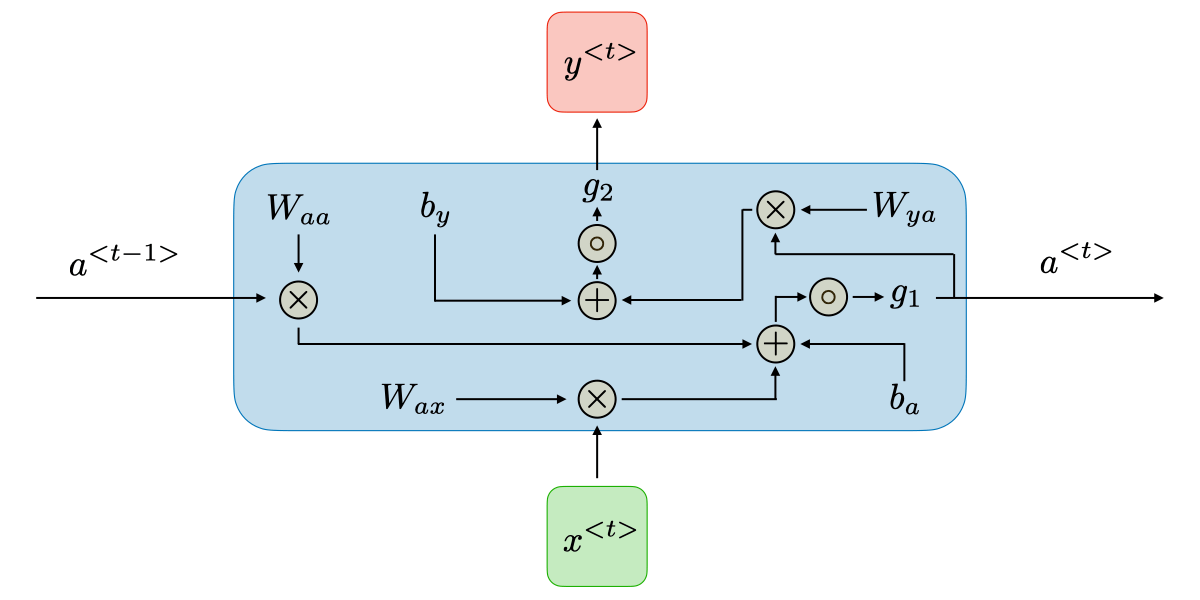### 损失函数$E$

$\mathcal{L}$ 为可微分的损失函数，比如交叉熵，其中$y^{<t>}$为t时刻正确的词语，$\hat{y}^{<t>}$为t时刻预测的词语。

$\mathcal{L}^{<t>} = \mathcal{L}(\hat{y}^{<t>}, y^{<t>}) \\ {E}(\hat{y}, y)=\sum_{t=1}^{T_{y}} \mathcal{L}^{<t>}$

### 反向传播## 总结

### 参考

posted @ 2021-01-25 12:05  段小辉  阅读(1089)  评论(0编辑  收藏  举报